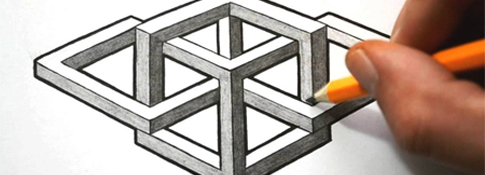##### Geometric Shapes #9###### Source: Listen In English

A simple drawing of geometric shapes including squares, cubes, circles, and vertical lines.###Directions

1. REVIEW the vocabulary.
2. LISTEN to the audio above.
3. DRAW the picture (on a piece of paper).

###Vocabulary

If you don't know any of the words below, check the Illustrated Dictionary.

• a circle
• a square
• a cube
• a triangle
• a line
• vertical
• upside down
• opaque
• transparent

###Script

This is a picture of four figures arranged horizontally across the page left to right.

The first figure on the left side of the page is a vertical line. In the middle of the vertical line, there is a small circle.

The second figure is a square. The height of the square is the same as the height of the first vertical line. In the middle of the square, there is a small triangle.

The third figure is a cube. The cube is about the same size as the square. The cube is opaque, so you can only see three sides of the cube - the front face, the top face and the right face.

In the middle of the front face of the cube, there is an upside down triangle. In the middle of the top face of the cube, there is a small circle. In the middle of the right face of the cube, there is a small square.

The fourth figure on the right side of the page is another cube. However, this cube is transparent, so you can see all of the lines. This cube is also about the same size as the square and the first cube.